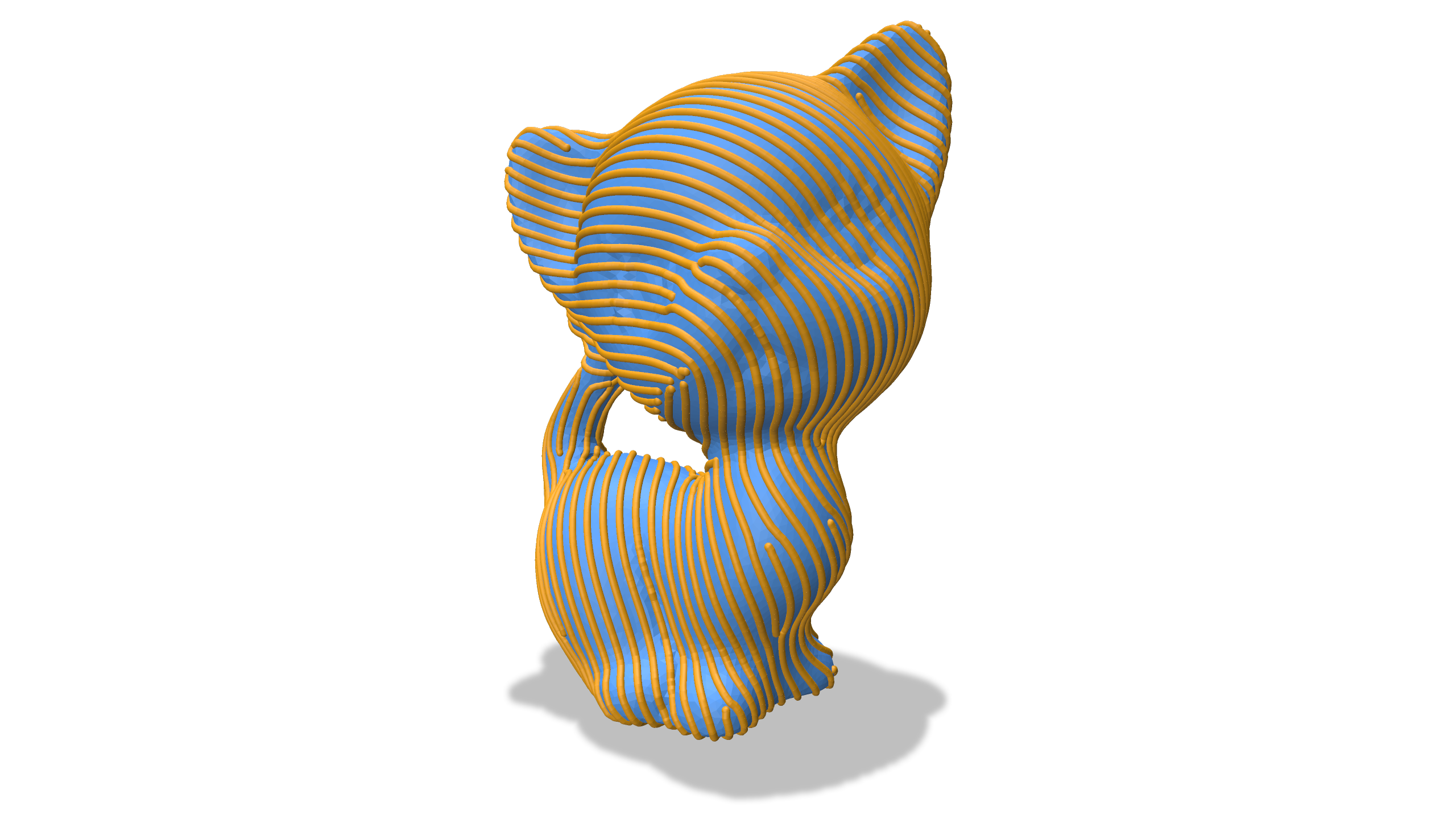# Stripes

The Stripe Patterns on Surfaces algorithm efficiently computes evenly-spaced stripes on a surface, aligned with some direction field given as input. This section describes an implementation in geometry-central, along with functions to extract the isolines of the stripes as polyline curves along the surface. The original reference implementation of the Stripes algorithm can be found here.

Authors: Original algorithm by Felix Knöppel, Keenan Cranel, Ulrich Pinkall, Peter Schröder. Extraction & geometry-central integration by David Jourdan.

#include "geometrycentral/surface/stripe_patterns.h"Example

// Generate a guiding field
VertexData<Vector2> guideField =
geometrycentral::surface::computeSmoothestVertexDirectionField(*geometry, 2);

// Compute the stripe pattern
dougle constantFreq = 40.;
VertexData<double> frequencies(*mesh, constantFreq);
CornerData<double> periodicFunc;
FaceData<int> zeroIndices;
FaceData<int> branchIndices;
std::tie(periodicFunc, zeroIndices, branchIndices) =
computeStripePattern(*geometry, frequencies, guideField);

// Extract isolines
std::vector<Vector3> isolineVerts;
std::vector<std::array<size_t, 2>> isolineEdges;
std::tie(isolineVerts, isolineEdges) = extractPolylinesFromStripePattern(
*geometry, periodicFunc, zeroIndices, branchIndices, guideField, false);


## Stripe Patterns

No surface interpolation

The Stripe Patterns paper describes a customized interpolation scheme to smoothly extend the scalar function on the interior of each triangle, even in the presence of singularities. That scheme is not yet implemented here; this implementation just computes a scalar function per-corner and extracts isolines. For the full scheme, see the original reference implementation.

std::tuple<CornerData<double>, FaceData<int>, FaceData<int>> computeStripePattern(IntrinsicGeometryInterface& geometry, const VertexData<double>& frequencies, const VertexData<Vector2>& directionField)

Compute a stripe pattern on the surface.

The direction field should be a 2-symmetric vector field, in the complex power representation.

std::tuple<std::vector<Vector3>, std::vector<std::array<size_t, 2>>> extractPolylinesFromStripePattern(EmbeddedGeometryInterface& geometry, const CornerData<double>& values, const FaceData<int>& stripeIndices, const FaceData<int>& fieldIndices, const VertexData<Vector2>& directionField, bool connectOnSingularities)

Process a stripe pattern ot extract isolines of the stripes as explicit polylines.

The direction field should be a 2-symmetric vector field, in the complex power representation.

Can optionally connect isolines separated by a singularity using a directionField alignment heuristic

std::tuple<std::vector<Vector3>, std::vector<std::array<size_t, 2>>> computeStripePatternPolylines(EmbeddedGeometryInterface& geometry, const VertexData<double>& frequencies, const VertexData<Vector2>& directionField, bool connectIsolinesOnSingularities = true)

Runs both of the above functions, computing the stripe pattern and extracting polylines from it.

The direction field should be a 2-symmetric vector field, in the complex power representation.

## Citation

These algorithms are described in Stripe Patterns on Surfaces

@article{Knoppel:2015:SPS,
author = {Kn\"{o}ppel, Felix and Crane, Keenan and Pinkall, Ulrich and Schr\"{o}der, Peter},
title = {Stripe Patterns on Surfaces},
journal = {ACM Trans. Graph.},
volume = {34},
issue = {4},
year = {2015},
publisher = {ACM},
address = {New York, NY, USA},
}